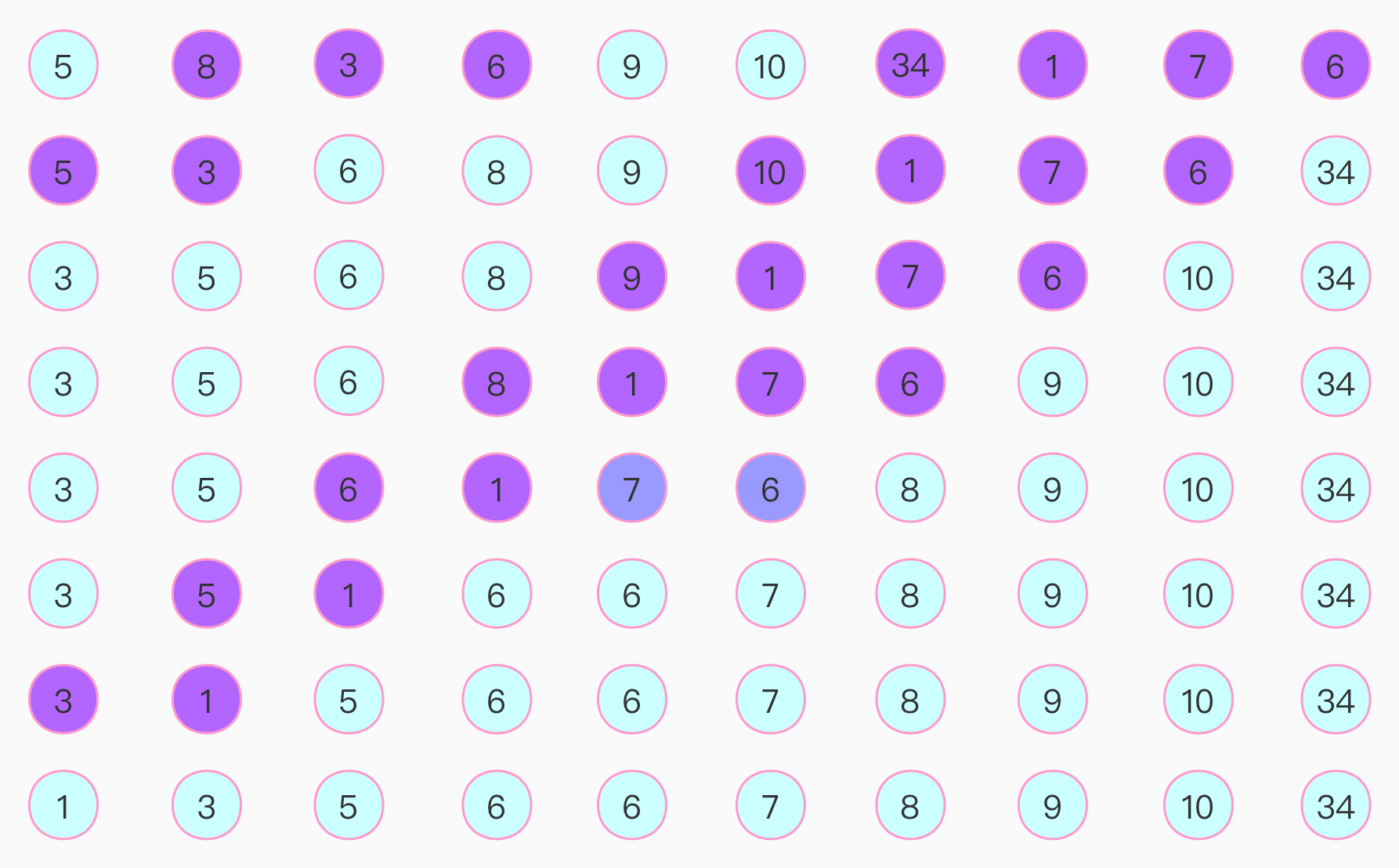# 算法-排序-冒泡排序

## 1.2 描述

• 比较相邻的元素。如果第一个比第二个大，就交换它们两个；

• 对每一对相邻元素作同样的工作，从开始第一对到结尾的最后一对，这样在最后的元素应该会是最大的数；

• 针对所有的元素重复以上的步骤，除了最后一个；

• 重复步骤1~3，直到排序完成。

## 1.3 代码

``````public class BubbleSort {
//    private static int[] number = {5, 8, 3, 6, 9, 10, 34, 1, 7, 12, 65, 4, 23, 2, 16, 15, 76, 56, 8};
private static int[] number = {5, 8, 3, 6, 9, 10, 34, 1, 7, 6};
private static String TAG = "BubbleSort";

public static void bubbleSort() {
int size = number.length;
int compareCount = 1;
Log.d(TAG, "sorted compare index 0 is " + Arrays.toString(number));
for (int i = 0; i < size - 1; i++) {
for (int j = 0; j < size - 1 - i; j++) {
if (number[j + 1] < number[j]) {
int temp = number[j + 1];
number[j + 1] = number[j];
number[j] = temp;
Log.d(TAG, "sorted compare index " + (compareCount++) + " is " + Arrays.toString(number));
}
}
}
for (int temp : number) {
Log.d(TAG, "sorted number is:" + temp);
}
}
}
``````

## 1.4 总结